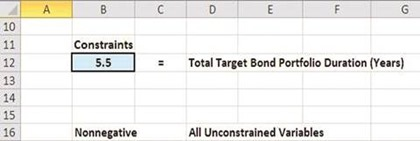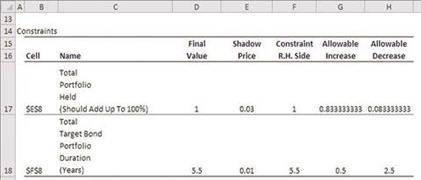# Optimizing the Allocation of Bonds in a Portfolio To Maximize Return

One basic use of the Solver is to correctly divide a bond portfolio among bonds of different yield, maturity, and risk or in order to maximize yield or minimize risk.

## The Problem

Correctly divide a bond portfolio among 4 bonds of varying yields and maturities in order to achieve an overall bond portfolio with an average maturity of 5.5 years while maximizing overall yield.

Specific information about each bond is as follows:(Click the Image To See a Larger Version)

## Excel Solver Problem Solving Steps

### Excel Solver Step 1 – Determine the Objective

In this case, the Objective is to create a portfolio that maximizes the overall portfolio yield while having a specific average maturity. The Objective is the overall yield of the entire portfolio. The cell that calculates this overall yield is the Objective Cell.

### Excel Solver Step 2 – Determine the Decision Variables

We are trying to determine what percentage of the overall portfolio to allocate to each bond in order to maximize the total yield while maintaining an average maturity of 5.5 years. The Decision Variables are the percentages of the overall portfolio that are allocated to bond type.

### Excel Solver Step 3 – Build the Excel Equations That Combine the Objective With All Decision Variables(Click the Image To See a Larger Version)

The yellow Objective cell G8 displays the overall portfolio yield and will be maximized. The green Decision Variable cells (E3 to E6) display the percentages of the overall portfolio to achieve the objective while creating a portfolio with an average maturity of 5.5 years. The light blue Constraint cell in the model (F8) establishes the average bond maturity and is controlled by the light blue user input in cell B12 in the following diagram:

### Excel Solver Step 4 – List All Constraints(Click the Image To See a Larger Version)

### Excel Solver Step 5 – Test the Excel Spreadsheet

Test the Excel spreadsheet completely before adding information to the Solver dialogue box. Make sure that any changes to green Decision Variables produce the correct results in the yellow Objective cell.

### Excel Solver Step 6 – Insert All Data into the Solver Dialogue Box

Input the Objective cell, Decision Variable cell, and all Constraints into the Solver dialogue box as follows:(Click the Image To See a Larger Version)

All equations on the Excel spreadsheet are linear (1st order) so we can use the Simplex LP (Linear Programming) method for this optimization problem.

Step 3 shows the completed problem with Decision Variables that have been optimized by the Solver to maximize the Objective while staying within the problem’s Constraints.

### Part 1

Note:

- The Solver Result

- How long Solver took to solve the problem

- The Solver Engine that was used and the Solver Options settings

- Where the Objective Cell was labeled in the Excel model for its name to appear as it does in Part 1 of the Answer Report(Click the Image To See a Larger Version)

### Part 2

- Note that the Variable Cells contain the Decision Variables

- Note where the labels for each Decision Variable are placed in the Excel model so that the Decision Variable’s name will appear here in Part 2 of the Answer Report as it does

- Note the type of variable - Either Continuous or Integer (Integer, Binary, or Alldifferent)

- Note the Before and After values of each Decision Variable(Click the Image To See a Larger Version)

### Part 3

- Note how each Constraint is labeled in the Excel model in order for the Constraint’s name to appear here in Part 3 of the Answer Report as it does

- Note which Constraints are binding (had their limits hit) and which aren’t.

- Note how much slack is still available in any Constraint that has not had its limit hit.

- Note any Integer Constraints (Integer, Binary, Alldifferent)(Click the Image To See a Larger Version)

## Excel Solver Limits Report

The Limits Report is made available when the Solver finds a Globally or Locally Optimal solution and no Integer Constraints (Integer, Binary, Alldifferent) were used. Part 2 of the Answer Report Shows that the variables were Continuous and not Integers. The Simplex LP method solves linear problems to globally optimal solutions.

The 2nd section of the Limits Report just shown indicates that none of the Decision Variables have any slack because the upper and lower limits of each Decision Variable are the same.(Click the Image To See a Larger Version)(Click the Image To See a Larger Version)

## Excel Solver Sensitivity Report

The Sensitivity Report is also made available when the Solver finds a Globally or Locally Optimal solution and no Integer Constraints (Integer, Binary, Alldifferent) were used. Part 2 of the Answer Report Shows that the variables were Continuous and not Integers. The Simplex LP method solves linear problems to globally optimal solutions.(Click the Image To See a Larger Version)(Click the Image To See a Larger Version)

Excel Master Series Blog Directory

Statistical Topics and Articles In Each Topic

• Histograms in Excel
• Bar Chart in Excel
• Combinations & Permutations in Excel
• Normal Distribution in Excel
• t-Distribution in Excel
• Binomial Distribution in Excel
• z-Tests in Excel
• t-Tests in Excel
• Hypothesis Tests of Proportion in Excel
• Chi-Square Independence Tests in Excel
• Chi-Square Goodness-Of-Fit Tests in Excel
• F Tests in Excel
• Correlation in Excel
• Pearson Correlation in Excel
• Spearman Correlation in Excel
• Confidence Intervals in Excel
• Simple Linear Regression in Excel
• Multiple Linear Regression in Excel
• Logistic Regression in Excel
• Single-Factor ANOVA in Excel
• Two-Factor ANOVA With Replication in Excel
• Two-Factor ANOVA Without Replication in Excel
• Randomized Block Design ANOVA in Excel
• Repeated-Measures ANOVA in Excel
• ANCOVA in Excel
• Normality Testing in Excel
• Nonparametric Testing in Excel
• Post Hoc Testing in Excel
• Creating Interactive Graphs of Statistical Distributions in Excel
• Solving Problems With Other Distributions in Excel
• Optimization With Excel Solver
• Chi-Square Population Variance Test in Excel
• Analyzing Data With Pivot Tables
• SEO Functions in Excel
• Time Series Analysis in Excel
• VLOOKUP

#### 1 comment:

1.Well, using MarketXLS works for me. It's great.﻿
Stock Portfolio Management in Excel